# Quantum Theory Quantum Theory based partly on Heisenbergs

• Slides: 15Quantum TheoryQuantum Theory (based partly on Heisenberg’s Uncertainty Principle) • The position and the momentum of a moving object cannot simultaneously be measured and known exactly • There is an inherent limitation to knowing both where a particle is at a particular moment and how it is moving in order to predict where it will be in the future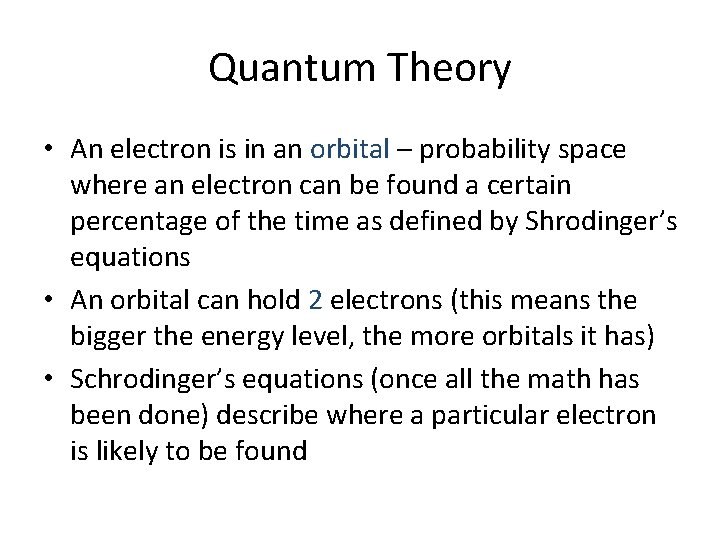Quantum Theory • An electron is in an orbital – probability space where an electron can be found a certain percentage of the time as defined by Shrodinger’s equations • An orbital can hold 2 electrons (this means the bigger the energy level, the more orbitals it has) • Schrodinger’s equations (once all the math has been done) describe where a particular electron is likely to be foundWe can know 4 pieces of information about the location of any electron within an atom. 1. Energy Level (n) – Distance from the nucleus – Options: 1 – 7 • 2. Sublevel (l) – Shape • 3. Magnetic Orientation (lm) – Direction the shape is pointing (think; x, y, z) • 4. Spin (ls) – How the electron is spinning (the options are up or down) – We write an up electron as a half arrow up ( ) and a down electron as a half arrow down ( )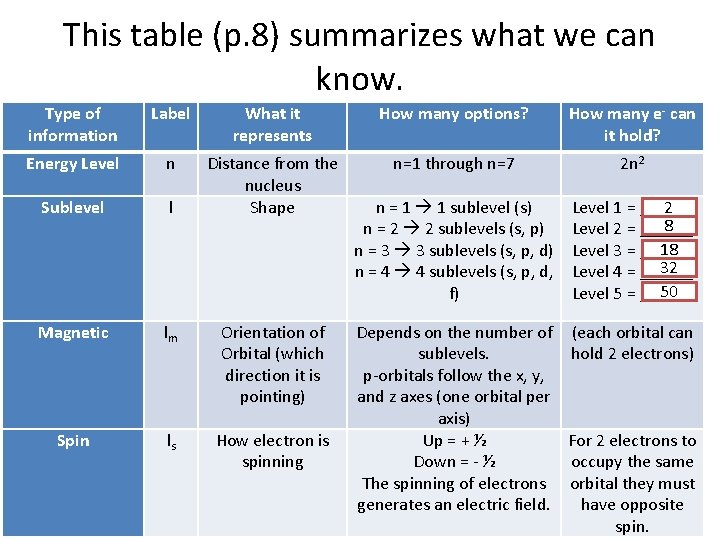This table (p. 8) summarizes what we can know. Type of information Label What it represents How many options? How many e- can it hold? Energy Level n n=1 through n=7 2 n 2 Sublevel l Distance from the nucleus Shape n = 1 1 sublevel (s) n = 2 2 sublevels (s, p) n = 3 3 sublevels (s, p, d) n = 4 4 sublevels (s, p, d, f) Level 1 = ______ 2 8 Level 2 = ______ 18 Level 3 = ______ 32 Level 4 = ______ 50 Level 5 = ______ Magnetic lm Orientation of Orbital (which direction it is pointing) Spin ls How electron is spinning Depends on the number of (each orbital can sublevels. hold 2 electrons) p-orbitals follow the x, y, and z axes (one orbital per axis) Up = + ½ For 2 electrons to Down = - ½ occupy the same The spinning of electrons orbital they must generates an electric field. have opposite spin.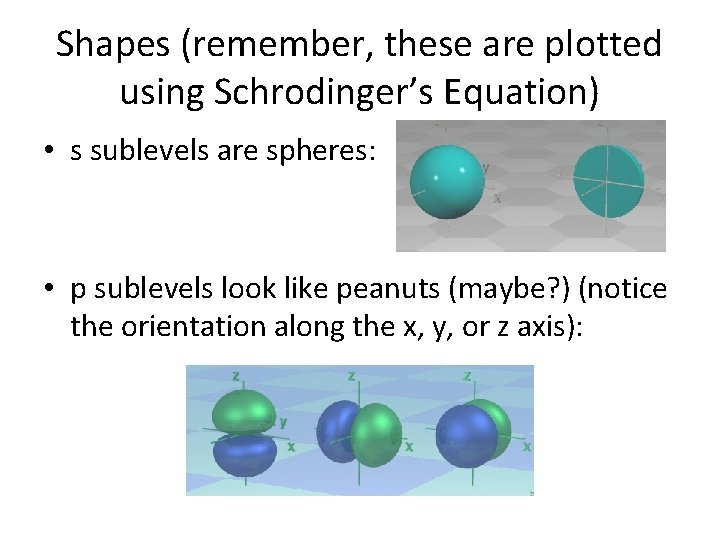Shapes (remember, these are plotted using Schrodinger’s Equation) • s sublevels are spheres: • p sublevels look like peanuts (maybe? ) (notice the orientation along the x, y, or z axis):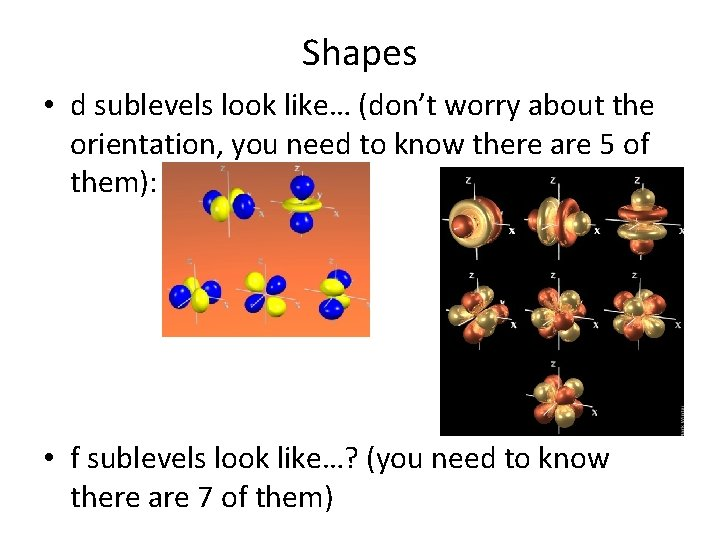Shapes • d sublevels look like… (don’t worry about the orientation, you need to know there are 5 of them): • f sublevels look like…? (you need to know there are 7 of them)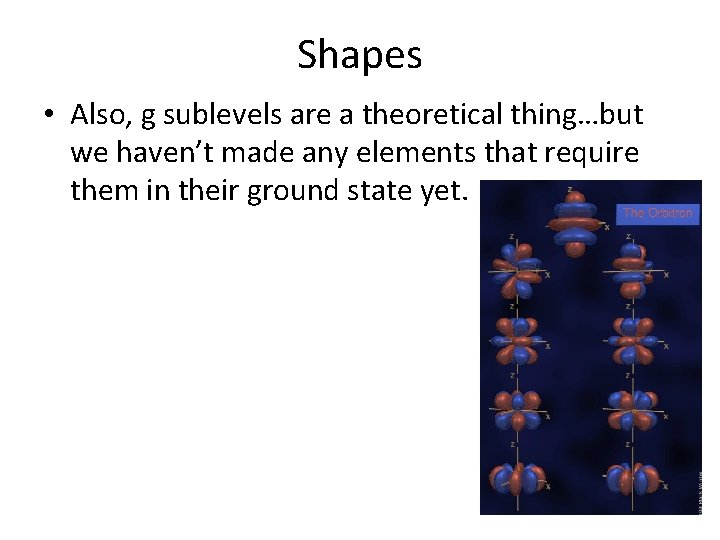Shapes • Also, g sublevels are a theoretical thing…but we haven’t made any elements that require them in their ground state yet.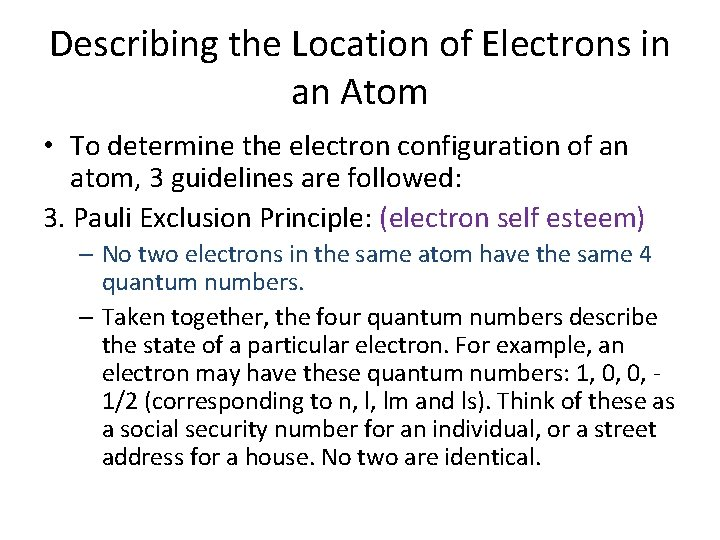Describing the Location of Electrons in an Atom • To determine the electron configuration of an atom, 3 guidelines are followed: 3. Pauli Exclusion Principle: (electron self esteem) – No two electrons in the same atom have the same 4 quantum numbers. – Taken together, the four quantum numbers describe the state of a particular electron. For example, an electron may have these quantum numbers: 1, 0, 0, 1/2 (corresponding to n, l, lm and ls). Think of these as a social security number for an individual, or a street address for a house. No two are identical.Describing the Location of Electrons in an Atom • To determine the electron configuration of an atom, 3 guidelines are followed: 1. Aufbau Principle: (electrons are lazy) – Electrons are added one at a time to the lowest energy available orbital. – 1 s is filled first, then 2 s, 2 p, 3 s, 3 p …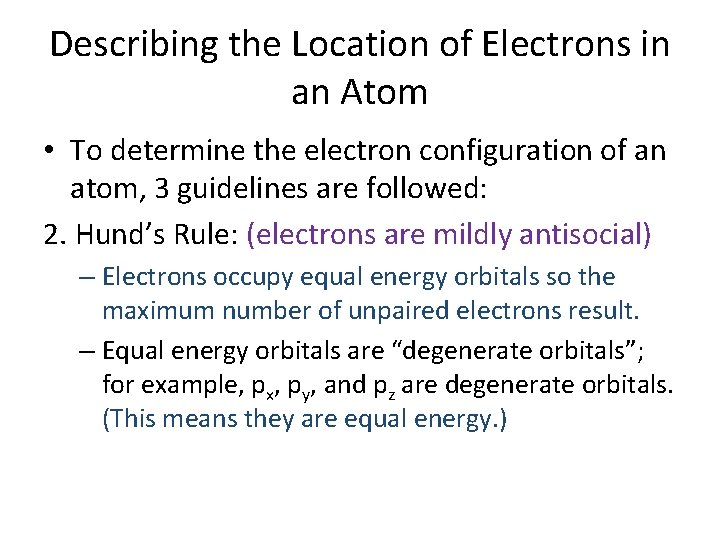Describing the Location of Electrons in an Atom • To determine the electron configuration of an atom, 3 guidelines are followed: 2. Hund’s Rule: (electrons are mildly antisocial) – Electrons occupy equal energy orbitals so the maximum number of unpaired electrons result. – Equal energy orbitals are “degenerate orbitals”; for example, px, py, and pz are degenerate orbitals. (This means they are equal energy. )Summary of energy levels, sublevels, and orbitals Principal Sublevel energy level Orbitals n=1 1 s 1 s (one) n=2 2 s, 2 p 2 s (one) + 2 p (three – x, y, z) n=3 3 s, 3 p, 3 d n=4 3 s (one) + 3 p (three – x, y, z) + 3 d (five – xy, xz, etc. ) 4 s, 4 p, 4 d, 4 f 4 s (one) + 4 p (three – x, y, z) + 4 d (five – xy, xz, etc. ) + 4 f (seven – xyz, etc. )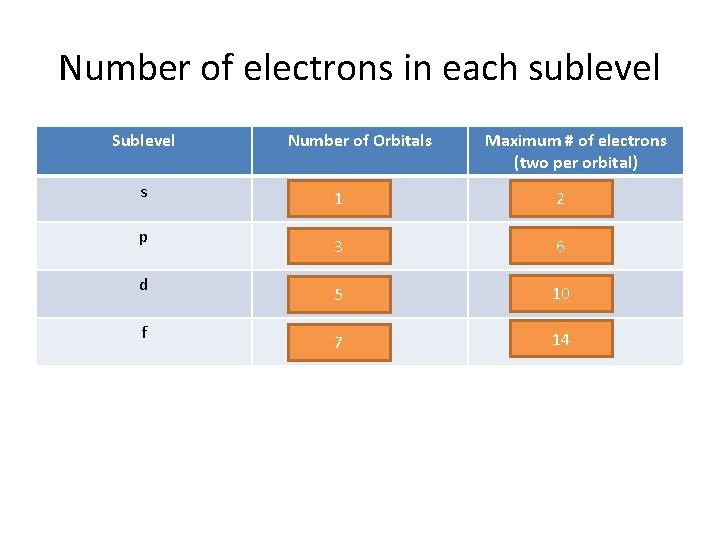Number of electrons in each sublevel Sublevel s p d f Number of Orbitals Maximum # of electrons (two per orbital) 1 2 3 6 5 10 7 14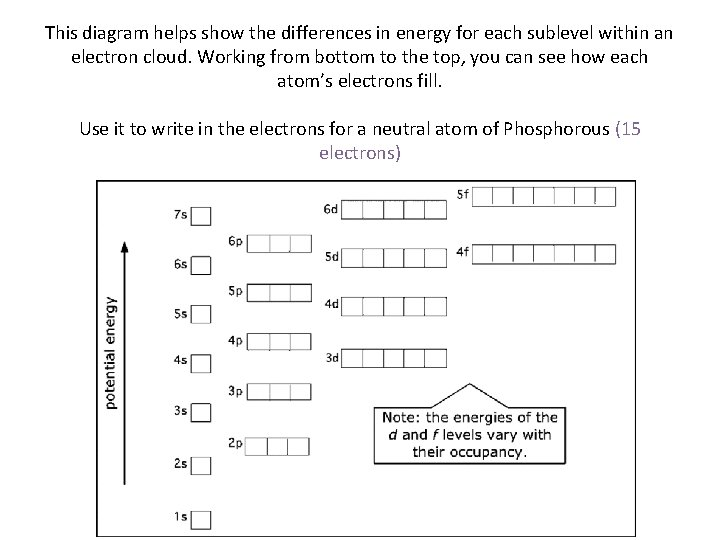This diagram helps show the differences in energy for each sublevel within an electron cloud. Working from bottom to the top, you can see how each atom’s electrons fill. Use it to write in the electrons for a neutral atom of Phosphorous (15 electrons)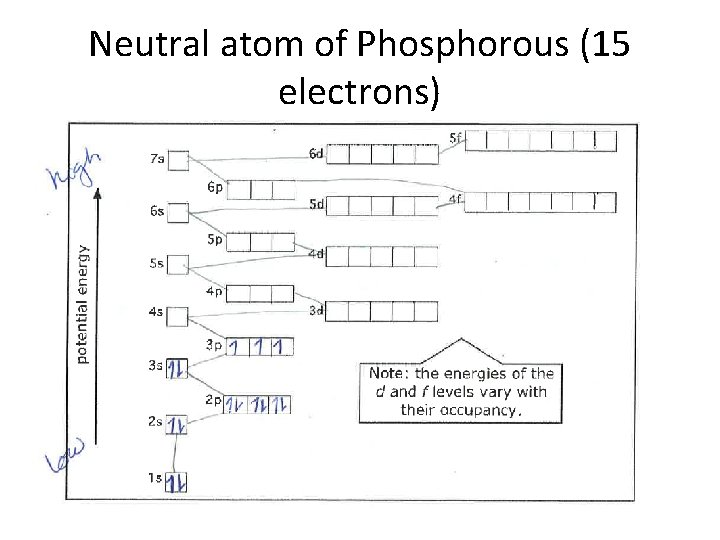Neutral atom of Phosphorous (15 electrons)